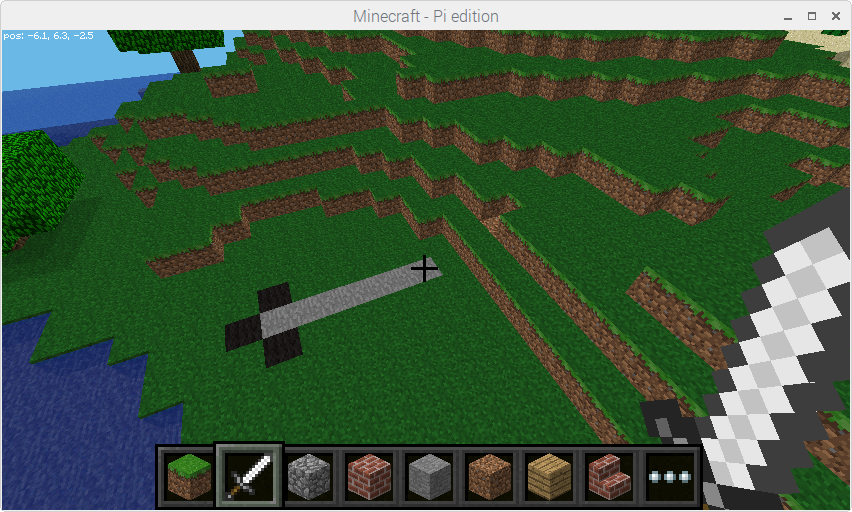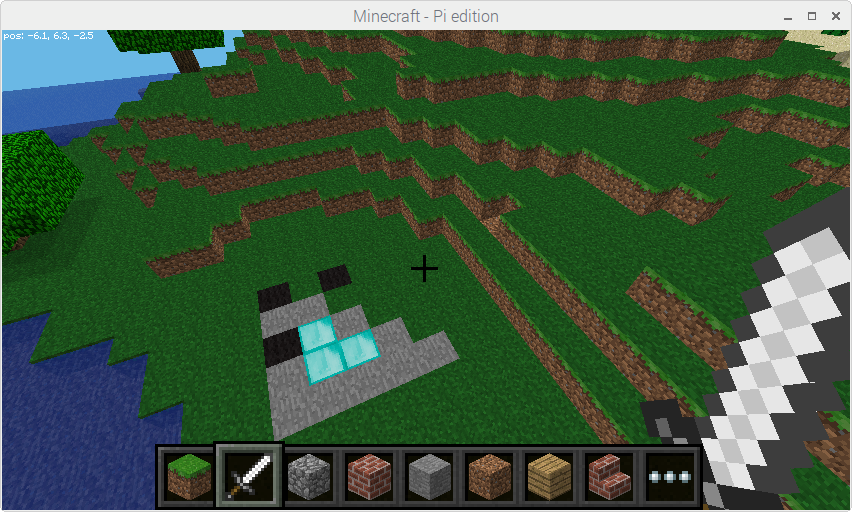# 4. Turtle Graphics¶

Turtle graphics is a popular way for introducing programming to kids. It was part of the original Logo programming language developed by Wally Feurzig and Seymour Papert in 1966.

The `turtle` module in Python’s standard library provides a classic implementation which moves a triangular turtle around a graphical window drawing geometric shapes.

The picraft turtle module is similar, but instead of a two-dimensional graphical window, its canvas is the Minecraft world. The module provides an object-oriented interface for advanced users that want to control multiple turtles and a simpler procedural interface for newer programmers.

When the turtle is initially created or shown, its default position is beneath the player’s feet:

```>>> from picraft.turtle import *
>>> showturtle()
```The turtle’s shape indicates its “forward” direction. Various simple commands can be used to control its orientation and motion:

```>>> right(180)
>>> forward(5)
```Every operation can be undone, and commands can be built up to construct whole shapes:

```>>> undo()
>>> penup()
>>> forward(5)
>>> left(90)
>>> pendown()
>>> fillblock('diamond_block')
>>> fill(True)
>>> forward(3)
>>> left(90)
>>> forward(4)
>>> left(135)
>>> forward(4)
>>> fill(False)
```## 4.1. Overview of available Turtle and TurtleScreen methods¶

### 4.1.1. Turtle methods¶

Turtle motion
Move and draw
Tell Turtle’s state
Pen control
Drawing state
Block control
Filling
More drawing control
Turtle state
Visibility
Special Turtle methods

## 4.2. Methods of Turtle and corresponding functions¶

### 4.2.1. Turtle motion¶

`picraft.turtle.``fd`(distance)
`picraft.turtle.``forward`(distance)
Parameters: distance (float) – the number of blocks to move forward.

Move the turtle forward by the specified distance, in the direction the turtle is headed:

```>>> position()
Vector(x=2, y=-1, z=13)
>>> forward(5)
>>> position()
Vector(x=2, y=-1, z=18)
>>> forward(-2)
>>> position()
Vector(x=2, y=-1, z=16)
```
`picraft.turtle.``back`(distance)
`picraft.turtle.``bk`(distance)
`picraft.turtle.``backward`(distance)
Parameters: distance (float) – the number of blocks to move back.

Move the turtle backward by the specified distance, opposite to the direction the turtle is headed. Does not change the turtle’s heading:

```>>> heading()
0.0
>>> position()
Vector(x=2, y=-1, z=18)
>>> backward(2)
>>> position()
Vector(x=2, y=-1, z=16)
0.0
```
`picraft.turtle.``lt`(angle)
`picraft.turtle.``left`(angle)
Parameters: angle (float) – the number of degrees to turn counter clockwise.

Turns the turtle left (counter-clockwise) by angle degrees:

```>>> heading()
90.0
>>> left(90)
0.0
```
`picraft.turtle.``rt`(angle)
`picraft.turtle.``right`(angle)
Parameters: angle (float) – the number of degrees to turn clockwise.

Turns the turtle right (clockwise) by angle degrees:

```>>> heading()
0.0
>>> right(90)
90.0
```
`picraft.turtle.``up`(angle)
Parameters: angle (float) – the number of degrees to increase elevation by.

Turns the turtle’s nose (its elevation) up by angle degrees:

```>>> elevation()
-45.0
>>> up(45)
>>> elevation()
0.0
```
`picraft.turtle.``dn`(angle)
`picraft.turtle.``down`(angle)
Parameters: angle (float) – the number of degrees to reduce elevation by.

Turns the turtle’s nose (its elevation) down by angle degrees:

```>>> elevation()
0.0
>>> down(45)
>>> elevation()
-45.0
```
`picraft.turtle.``setpos`(x, y=None, z=None)
`picraft.turtle.``setposition`(x, y=None, z=None)
`picraft.turtle.``goto`(x, y=None, z=None)
Parameters: x (float) – the new x coordinate or a turtle / triple / `Vector` of numbers y (float) – the new y coordinate or `None` z (float) – the new z coordinate or `None`

Moves the turtle to an absolute position. If the pen is down, draws a line between the current position and the newly specified position. Does not change the turtle’s orientation:

```>>> tp = pos()
>>> tp
Vector(x=2, y=-1, z=16)
>>> setpos(4, -1, 16)
>>> pos()
Vector(x=4, y=-1, z=16)
>>> setpos((0, -1, 16))
>>> pos()
Vector(x=0, y=-1, z=16)
>>> setpos(tp)
>>> pos()
Vector(x=2, y=-1, z=16)
```

If y and z are `None`, x must be a triple of coordinates, a `Vector`, or another Turtle.

`picraft.turtle.``setx`(x)
Parameters: x (float) – the new x coordinate

Set the turtle’s first coordinate to x; leave the second and third coordinates unchanged:

```>>> position()
Vector(x=2, y=-1, z=16)
>>> setx(5)
>>> position()
Vector(x=5, y=-1, z=16)
```
`picraft.turtle.``sety`(y)
Parameters: y (float) – the new y coordinate

Set the turtle’s second coordinate to y; leave the first and third coordinates unchanged:

```>>> position()
Vector(x=2, y=-1, z=16)
>>> sety(5)
>>> position()
Vector(x=2, y=5, z=16)
```
`picraft.turtle.``setz`(z)
Parameters: z (float) – the new z coordinate

Set the turtle’s third coordinate to z; leave the first and second coordinates unchanged:

```>>> position()
Vector(x=2, y=-1, z=16)
>>> setz(5)
>>> position()
Vector(x=2, y=-1, z=5)
```
`picraft.turtle.``seth`(to_angle)
`picraft.turtle.``setheading`(to_angle)
Parameters: to_angle (float) – the new heading

Set the orientation of the turtle on the ground plane (X-Z) to to_angle. The common directions in degrees correspond to the following axis directions:

0 +Z
90 +X
180 -Z
270 -X
```>>> setheading(90)
90.0
```
`picraft.turtle.``sete`(to_angle)
`picraft.turtle.``setelevation`(to_angle)
Parameters: to_angle (float) – the new elevation

Set the elevation of the turtle away from the ground plane (X-Z) to to_angle. At 0 degrees elevation, the turtle moves along the ground plane (X-Z). At 90 degrees elevation, the turtle moves vertically upward, and at -90 degrees, the turtle moves vertically downward:

```>>> setelevation(90)
>>> elevation()
90.0
```
`picraft.turtle.``home`()

Move the turtle to its starting position (this is usually beneath where the player was standing when the turtle was spawned), and set its heading to its start orientation (0 degrees heading, 0 degrees elevation):

```>>> heading()
90.0
>>> elevation
45.0
>>> position()
Vector(x=2, y=-1, z=16)
>>> home()
>>> position()
Vector(x=0, y=-1, z=0)
0.0
>>> elevation()
0.0
```
`picraft.turtle.``undo`()

Undo (repeatedly) the last turtle action(s):

```>>> for i in range(4):
...     fd(5)
...     lt(90)
...
>>> for i in range(8):
...     undo()
```

### 4.2.2. Tell Turtle’s state¶

`picraft.turtle.``position`()
`picraft.turtle.``pos`()

Return the turtle’s current location (x, y, z) as a `Vector`:

```>>> pos()
Vector(x=2, y=-1, z=18)
```
`picraft.turtle.``towards`(x, y=None, z=None)
Parameters: x (float) – the target x coordinate or a turtle / triple / `Vector` of numbers y (float) – the target y coordinate or `None` z (float) – the target z coordinate or `None`

Return the angle between the line from the turtle’s position to the position specified within the ground plane (X-Z):

```>>> home()
>>> forward(5)
>>> towards(0, 0, 0)
-180.0
>>> left(90)
>>> forward(5)
>>> towards(0, 0, 0)
135.0
```

If y and z are `None`, x must be a triple of coordinates, a `Vector`, or another Turtle.

`picraft.turtle.``heading`()

Return the turtle’s current heading (its orientation along the ground plane, X-Z):

```>>> home()
>>> right(90)
90.0
```
`picraft.turtle.``elevation`()

Return the turtle’s current elevation (its orientation away from the ground plane, X-Z):

```>>> home()
>>> up(90)
>>> elevation()
90.0
```
`picraft.turtle.``xcor`()

Return the turtle’s x coordinate:

```>>> home()
>>> xcor()
0
>>> left(90)
>>> forward(2)
>>> xcor()
2
```
`picraft.turtle.``ycor`()

Return the turtle’s y coordinate:

```>>> home()
>>> ycor()
-1
>>> up(90)
>>> forward(2)
>>> ycor()
1
```
`picraft.turtle.``zcor`()

Return the turtle’s z coordinate:

```>>> home()
>>> zcor()
0
>>> forward(2)
>>> zcor()
2
```
`picraft.turtle.``distance`(x, y=None, z=None)
Parameters: x (float) – the target x coordinate or a turtle / triple / `Vector` of numbers y (float) – the target y coordinate or `None` z (float) – the target z coordinate or `None`

Return the distance from the turtle to (x, y, z), the given vector, or the given other turtle, in blocks:

```>>> home()
>>> distance((0, -1, 5))
5.0
>>> forward(2)
>>> distance(0, -1, 5)
3.0
```

## 4.3. Pen control¶

### 4.3.1. Drawing state¶

`picraft.turtle.``pd`()
`picraft.turtle.``pendown`()

Put the “pen” down; the turtle draws new blocks when it moves.

`picraft.turtle.``pu`()
`picraft.turtle.``penup`()

Put the “pen” up; movement doesn’t draw new blocks.

`picraft.turtle.``isdown`()

Returns `True` if the pen is down, `False` if it’s up.

### 4.3.2. Block control¶

`picraft.turtle.``penblock`(*args)

Return or set the block that the turtle draws when it moves. Several input formats are allowed:

`penblock()`
Return the current pen block. May be used as input to another penblock or fillblock call.
`penblock(Block('grass'))`
Set the pen block to the specified `Block` instance.
`penblock('grass')`
Implicitly make a `Block` from the given arguments and set that as the pen block.
```>>> penblock()
<Block "stone" id=1 data=0>
>>> penblock('diamond_block')
>>> penblock()
<Block "diamond_block" id=57 data=0>
>>> penblock(1, 0)
>>> penblock()
<Block "stone" id=1 data=0>
```
`picraft.turtle.``fillblock`(*args)

Return or set the block that the turtle fills shapes with. Several input formats are allowed:

`fillblock()`
Return the current fill block. May be used as input to another penblock or fillblock call.
`fillblock(Block('grass'))`
Set the fill block to the specified `Block` instance.
`fillblock('grass')`
Implicitly make a `Block` from the given arguments and set that as the fill block.
```>>> fillblock()
<Block "stone" id=1 data=0>
>>> fillblock('diamond_block')
>>> fillblock()
<Block "diamond_block" id=57 data=0>
>>> fillblock(1, 0)
>>> fillblock()
<Block "stone" id=1 data=0>
```

### 4.3.3. Filling¶

`picraft.turtle.``fill`(flag=None)
Parameters: flag (bool) – True if beginning a fill, False if ending a fill.

Call `fill(True)` before drawing the shape you want to fill, and `fill(False)` when done. When used without argument: return the fill state (`True` if filling, `False` otherwise).

`picraft.turtle.``begin_fill`()

Call just before drawing a shape to be filled. Equivalent to `fill(True)`.

`picraft.turtle.``end_fill`()

Fill the shape drawn after the last call to `begin_fill()`. Equivalent to `fill(False)`.

### 4.3.4. More drawing control¶

`picraft.turtle.``reset`()
`picraft.turtle.``clear`()

## 4.4. Turtle state¶

### 4.4.1. Visibility¶

`picraft.turtle.``st`()
`picraft.turtle.``showturtle`()

Make the turtle visible:

```>>> showturtle()
```
`picraft.turtle.``ht`()
`picraft.turtle.``hideturtle`()

Make the turtle invisible:

```>>> hideturtle()
```
`picraft.turtle.``isvisible`()

Return `True` if the turtle is shown, `False` if it’s hidden:

```>>> hideturtle()
>>> isvisible()
False
>>> showturtle()
>>> isvisible()
True
```

## 4.5. Special Turtle methods¶

`picraft.turtle.``undobufferentries`()

Return number of entries in the undobuffer:

```>>> while undobufferentries():
...     undo()
```
`picraft.turtle.``getpen`()
`picraft.turtle.``getturtle`()

Return the `Turtle` object itself. Only reasonable use: as a function to return the “anonymous” turtle:

```>>> pet = getturtle()
>>> pet.fd(50)
>>> pet
<picraft.turtle.Turtle object at 0x...>
```
`picraft.turtle.``getscreen`()

Return the `TurtleScreen` object the turtle is drawing on:

```>>> ts = getscreen()
>>> ts
<picraft.turtle.TurtleScreen object at 0x...>
>>> ts.world.say("Hello world!")
```